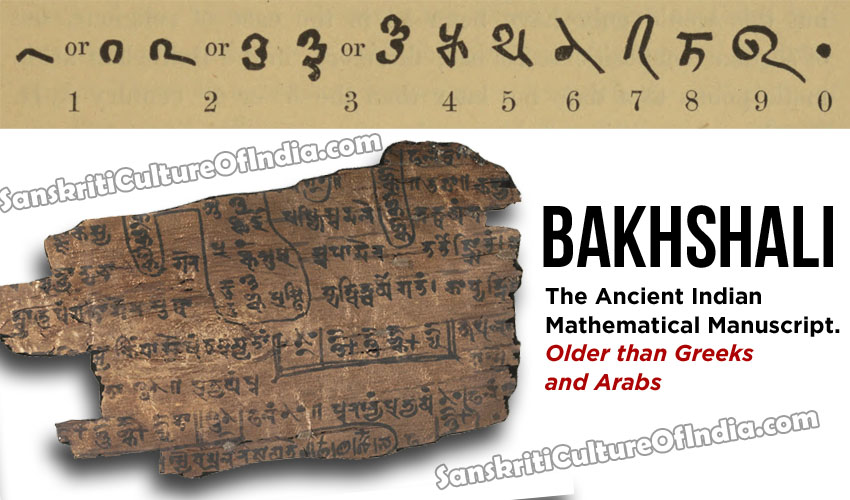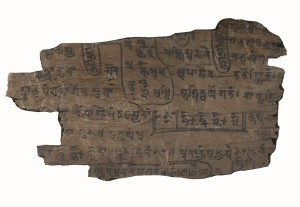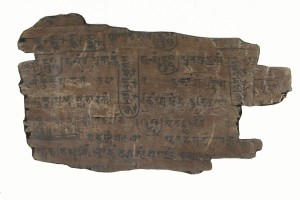IndianSanskritiBakhshali: The Ancient Indian Mathematical Manuscript

The Bakhshali Manuscript is an Ancient Indian mathematical manuscript written on “birch bark” which was found near the village of Bakhshali in 1881 in what was then the North-West Frontier Province of British India (now Khyber Pakhtunkhwa province, now in Pakistan).Bakhshali Manuscript is written in Śāradā script and in Gatha dialect (which is a combination of the ancient Indian languages of Sanskrit and Prakrit). The manuscript is incomplete, with only seventy leaves of birch bark, many of which are mere scraps. Many remain undiscovered. The Bakhshali manuscript, which is currently too fragile to be examined by scholars, is currently housed in the Bodleian Library at the University of Oxford and is too fragile to be examined by scholars.

It does not appear to belong to any specific period. Although that said, G. Joseph classes it as a work of the early ‘classical period’, while E Robertson and J O’Connor suggest it may be a work of Jain mathematics, and while this is chronologically plausible there is no proof it was composed by Jain scholars. L Gurjar discusses its date in detail, and concludes it can be dated no more accurately than ‘between 2nd century BC and 2nd century AD’. He offers compelling evidence by way of detailed analysis of the contents of the manuscript (originally carried by R Hoernle). His evidence includes the language in which it was written (‘died out’ around 300 AD), discussion of currency found in several problems, and the absence of techniques known to have been developed by the 5th century.

Historians who have placed the date at pre 450 AD and identified the ‘current’ version as a copy.

Avoiding further debate, L Gurjar states that the Bakshali manuscript is the:

…Capstone of the advance of mathematics from the Vedic age up to that period…

Although, as much work was lost between ‘periods’, we cannot fully gauge continuity of progress and it is possible the composer(s) of the Bakhshali manuscript were not fully aware of earlier works and had to start from ‘scratch’. This would make the work an even more remarkable achievement.

The arithmetic contained within the work is of such a high quality that it has been suggested:

…In fact [the] Greeks [are] indebted to India for much of the developments in Arithmetic…

Some of the contents of Manuscript are elaborated here.

Examples of the rule of three (and profit and loss and interest). Solution of linear equations with as many as five unknowns. The solution of the quadratic equation (development of remarkable quality). Arithmetic (and geometric) progressions. Compound Series (some evidence that work begun by Jains continued). Quadratic indeterminate equations (origin of type ax/c = y). Simultaneous equations.Fractions and other advances in notation including use of zero and negative sign. Improved method for calculating square root (and hence approximations for irrational numbers). The improved method (shown below) allowed extremely accurate approximations to be calculated:

A = (a2 + r) = a + r/2a – {(r/2a)2 / 2(a + r/2a)}

Example 6.1: Application of square root formula.
Again we can calculate 10, where a = 3 and r = 1.10 = (32 + 1) = 3 + 1/6 – {(1/36)/2(3 + 1/6)}= 3 + 1/6 – {(1/36)/(19/3)}= 3 + 1/6 – 1/228= 3.16228… in decimal form root(10) = 3.16228 when calculated on a calculator and rounded to five decimal places.

Example 6.2: Quadratic equation as found in B. Ms.
If the equation given is dn2 + (2a – d)n -2s = 0 then the solution is found using the equation:
n = (- (2a – d) (2a – d)2 +8ds))/2dWhich is the quadratic equation with a = d, b = 2a – d, and c = 2s.

Example 6.3: Linear equation with 5 variables.
The following problem is stated : ”Five merchants together buy a jewel. Its price is equal to half the money possessed by the first together with the money possessed by the others, or one-third the money possessed by the second together with the moneys of the others, or one-fourth the money possessed by the third together with the moneys of the others…etc. Find the price of the jewel and the money possessed by each merchant.

Solution : We have the following systems of equations:
x1/2 + x2 + x3 + x4 + x5
= px1 + x2/3 + x3 + x4 + x5
= px1 + x2 + x3/4 + x4 + x5
= px1 + x2 + x3 + x4/5 +x5
= px1 + x2 + x3 + x4 + x5/6 = p

Then if x1/2 + x2/3 + x3/4 + x4/5 + x5/6 = q ,
the equations become (377/60 )q = p.A number of possible answers can be obtained. This is the origin of the indeterminate equation of the type ax/c = y, the theory of which was greatly developed, and later perfected by Bhaskara II, four hundred years before it was discovered in Europe. If q = 60 then p = 377 and x1 = 120, x2 = 90, x3 = 80, x4 = 75 and x5 = 72

Historians of mathematics debate whether true algebra ‘began’ in Greece or Arabia, and little mention is ever made of Indian algebra. In light of my own research I feel that early Arabic algebra (c. 800 AD) in no way surpasses the level of understanding of 6th century Indian scholars.

The Bakhshali manuscript is a unique piece of work and while it not only contains mathematics of a remarkably high standard for the time period, also, in contrast to almost all other Indian works composed before and after, the method of the commentary follows a highly systematic order of:

i. Statement of the rule (sutra)
ii. Statement of the examples (udaharana)
iii. Demonstration of the operation (karana) of the rule.

By the end of the 2nd century AD mathematics in India had attained a considerable stature, and had become divorced from purely practical and religious requirements, (although it is worth noting that over the next 1000 years the majority of mathematical developments occurred within works on astronomy).

The topics of algebra, arithmetic and geometry had developed significantly and it is widely thought that the decimal place value system of notation had been (generally) perfected by 200 AD, the consequence of which was far reaching.

~ Ian Pearce

This site uses Akismet to reduce spam. Learn how your comment data is processed.

•Stephen says:

i would like to know the author of this article, is it ‘ Ian Pearce’ – there are so many Ian Pearce on the google search that the one with this article seem remote, I have a contention with a peer that the world oldest university was in India.

•Vyom Jayaswal says:

Yes, that’s right. It was Nalanda University.

•sharath says:

indeed the world’s oldest university was in india , the muslim invaders destroyed it and it was told that they took weeks to burn the books from the university which housed around 2000 students around the world

•ABHISHEK Goswami says:

First university of world :
Takhshhshila ( taxila)
In Indus valley civilization ( off spring of saraswati river civilization)
Mathematics
Astrology
Medical science
Philosophy
All Arabians and Greek learned from here.
Democrotos learned from here.
Taxila university was established in 700 bce.

• […] must also try to get the dates of the Bakhsahali manuscript agreed to find the ownership of the first available zero India was the first to discover zero. The […]

•Prasad says:

Why dont we put in front of the world that all these were already discovered by us loong back….

•Anand says:

We have don’t worry see G a tularam google

Pseudo Secularism hurting India!!